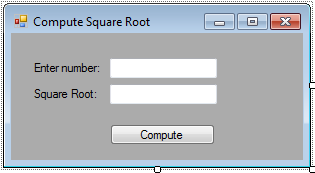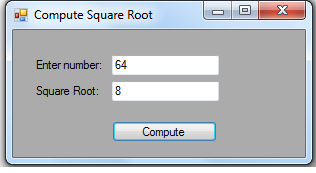# Compute Square Root using Math.Sqrt in VB.NET

In this article, i will introduce another function in VB.NET regarding the Math Functions, the Math.Sqrt(). Math.Sqrt() function provides to compute the square root of a number. This will help the students to solve their quizzes and exams regarding how to get a square root of a number by only a manual solving. haha. LOL. :)

So, now let's start this tutorial!

1. Let's start with creating a Windows Form Application for this tutorial by following the following steps in Microsoft Visual Studio: Go to File, click New Project, and choose Windows Application.

2. Next, add two TextBoxes named TextBox1 and TextBox2 then leave it as blank. TextBox1 will be your whole number as an input, and TextBox2 will be the result of the square root you've input. Now, add a Button named Button1 to see the result of the Square Root and displayed in TextBox2. You must design your layout like this:3. Now, put this code in Button1_Click. This will display the result of the square root in TextBox2.

` Private Sub Button1_Click(ByVal sender As System.Object, ByVal e As System.EventArgs) Handles Button1.Click        Dim num1 As Integer        num1 = Val(TextBox1.Text)        TextBox2.Text = Math.Sqrt(num1)    End Sub`

We initialized num1 as Integer to hold the value inputted in TextBox1. Then in TextBox2, we compute the square root of a number inputted in num1 with the syntax `Math.Sqrt(num1)`. Math.Sqrt() Function is only for calculating the square root of number.

#### Output:For more inquiries and need programmer for your thesis systems in any kind of programming languages, just contact my number below.

Best Regards,

Engr. Lyndon R. Bermoy
IT Instructor/System Developer/Android Developer
STI College - Surigao City
Mobile: 09488225971
E-mail:[email protected]

Note: Due to the size or complexity of this submission, the author has submitted it as a .zip file to shorten your download time. After downloading it, you will need a program like Winzip to decompress it.

Virus note: All files are scanned once-a-day by SourceCodester.com for viruses, but new viruses come out every day, so no prevention program can catch 100% of them.